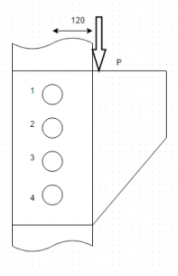Courses

# Test: Eccentrically Loaded Riveted Joints

## 9 Questions MCQ Test Machine Design | Test: Eccentrically Loaded Riveted Joints

Description
This mock test of Test: Eccentrically Loaded Riveted Joints for Mechanical Engineering helps you for every Mechanical Engineering entrance exam. This contains 9 Multiple Choice Questions for Mechanical Engineering Test: Eccentrically Loaded Riveted Joints (mcq) to study with solutions a complete question bank. The solved questions answers in this Test: Eccentrically Loaded Riveted Joints quiz give you a good mix of easy questions and tough questions. Mechanical Engineering students definitely take this Test: Eccentrically Loaded Riveted Joints exercise for a better result in the exam. You can find other Test: Eccentrically Loaded Riveted Joints extra questions, long questions & short questions for Mechanical Engineering on EduRev as well by searching above.
QUESTION: 1

###A bracket is attached to a vertical wall by means of four rivets. Find the rivet which is under maximum stress.

Solution:

Explanation: Primary Shear act vertically upward on all the rivets. Secondary shear is proportional to the distance from CG. Hence rivets 1 and 4 are under maximum stress.

QUESTION: 2

###Calculate the primary shear stress on each rivet if P=30kN and diameter of rivets is 15mm.

Solution:

Explanation: τ₁=P/4A or 7500/A.

QUESTION: 3

### If secondary shear stress acting on any bolts is given by Cxr₁ where r₁ is the distance of bolt from CG, then find the value of C. Bolts are equidistant with spacing of 100mm. Force P=30kN.

Solution:

Explanation: C=Pe/[r₁²+r₂²+r₃²+r₄²].

QUESTION: 4

Calculate the effective force in vector form to which rivet 2 is subjected. Bolts are separated by 100mm and force P=30kN.

Solution:

Explanation: Primary force=7500j, Sec Force=Cx50(-i) where C=7

QUESTION: 5

Calculate the effective force in vector form to which rivet 4 is subjected. Bolts are separated by 100mm and force P=30kN.

Solution:

Explanation: Primary force=7500j, Sec Force=Cx100 i where C=72.

QUESTION: 6

Calculate the diameter of the rivets if permissible shear stress is 60N/mm². Bolts are separated by 100mm and force P=30kN.

Solution:

Explanation: Magnitude of force acting on Bolt 4 or Bolt 1=√7500²+7200² or 10396.6=πd²τ/4.

QUESTION: 7

Are the bolts 2 and 3 under same force?

Solution:

Explanation: Primary shear force is same but secondary shear forces direction are different in the two bolts.

QUESTION: 8

Are the bolts 2 and 3 subjected to same magnitude of force?

Solution:

Explanation: Although the vectors are different for the forces on two bolts but their magnitudes are same.

QUESTION: 9

Can we use the rivets of diameter 18mm in the following case if P=30kN and bolts are separated by 100mm each. Maximum permissible shear stress is 60N/mm².

Solution:

Explanation: The minimum diameter required for the rivets is obtained 15mm. Any rivet greater than this diameter is safe to use.